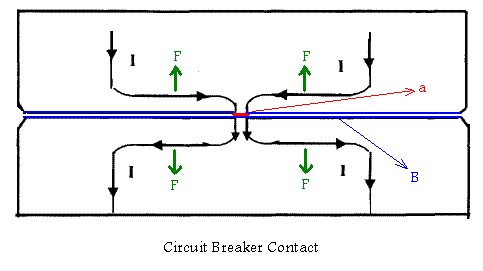Home » Topics » English riddles » General Subjects » Electrical riddle No.37 – High current circuit breakers

# Electrical riddle No.37 – High current circuit breakers

• This topic has 1 reply, 1 voice, and was last updated 1 year, 8 months ago byHamid.
• Creator
Topic
• #309Mohammed

Why strong spring usually is used in high current circuit breakers? Why required forces for operation of circuit breaker shall be increased when its rated current or short circuit capacity is increased?

Viewing 1 replies (of 1 total)
• Author
Replies
• #1591Hamid

1-For overcome of repulsion forces of circuit breaker contacts due to high through fault currents 2-For reduction of repulsion forces between moving electrical contacts of circuit breaker As you know, fundamentally there is a current constriction at the point of electrical contact. We also know that this constriction is responsible for the contact resistance and consequently for the heat being generated at the contacts, but in addition to this the current constriction is also the source of electromagnetic forces acting upon the contact structures. In below Figure the current path of the current at the mating surface of the contact is shown.As it can be seen from the figure, as the current constricts into a transfer point there is a component of the current that flows in opposite directions and thus the net result is a repelling force trying to force apart the contacts. The repulsion force is given by: F=10-7. I2 . ln (B/a) Newton Where: B = Contact area a = Actual area of contact point With increasing of electrical contact pressures, the concentrated current area (a) can be increased which cause the reduction of repulsion forces. It is difficult however to properly use this formula because of the difficulty in defining the actual contact area. For practical purposes some expressions that yield adequate results have been proposed. For example: F= 0.115 (I/n)2 lb.per finger Or practical relationship which was obtained experimentally with a 3 inch Cu-Cr butt type contact in vacuum is given by the expression: F= 0.885 (I/n)1.51 lb.per finger Where: I = Peak current in kA n = Number of contacts The differences on the force requirements obtained depending on which expression is used serve to point out the uncertainty of the variables involved and the probabilistic nature of the forces. In general the application of higher forces would yield a higher confidence level and a higher probability of withstanding the repulsion forces.

Viewing 1 replies (of 1 total)
• You must be logged in to reply to this topic.